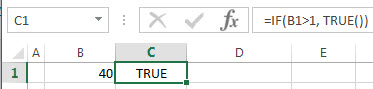# Excel TRUE Function

This post will guide you how to use Excel TRUE function with syntax and examples in Microsoft excel.

### Description

The Excel TRUE function return the logical value TRUE. And you can use the TRUE function when you want to return the TRUE value. For example, the formula: =IF(B1>5, TRUE()).

The TRUE function is a build-in function in Microsoft Excel and it is categorized as a Logical Function.

The TRUE function is available in Excel 2016, Excel 2013, Excel 2010, Excel 2007, Excel 2003, Excel XP, Excel 2000, Excel 2011 for Mac.

### Syntax

The syntax of the TRUE function is as below:

`=TRUE ()`

There are no parameters or arguments for the TRUE function.

### Excel TRUE Function Examples

The below examples will show you how to use Excel TRUE Function to return the logical value TRUE

If you want to get the TRUE value, you just need to enter the word TRUE directly in to a cell or formula or you can enter the TRUE() function into a formula. And then it will be interpret as the logical value TRUE. Let’s see the below two formulas:

=IF(B1>1, TRUE())

or

=IF(B1>1, TRUE)Related Functions

• Excel FALSE function
The Excel FALSE function returns the logical value “FALSE”.The FALSE function is a build-in function in Microsoft Excel and it is categorized as a Logical Function.The syntax of the FALSE function is as below:= FALSE ()…